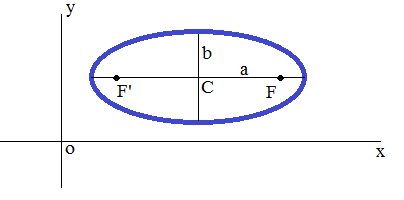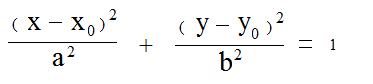## Ellipse Equation Calculator

 x0 : y0 : a : b :
Ellipse Focus F:
Ellipse Focus F':
Ellipse Eccentricity :
Area :
Circumference :
Center to Focus Distance :

Ellipse equation and graph with center C(x0, y0) and major axis parallel to x axis. If the major axis is parallel to the y axis, interchange x and y during your calculation.Ellipses have two mutually perpendicular axes about which the ellipse is symmetric. These axes intersect at the center of the ellipse due to this symmetry. The larger of these two axes, which corresponds to the largest distance between antipodal points on the ellipse, is called the major axis or transverse diameter.The calculation is made easier here.

Thinkcalculator.com provides you helpful and handy calculator resources.